# Lowering in Vapour Pressure Assignment Help## Lowering in Vapour Pressure

When a non volatile solute is present in the solution, the vapour pressure of the solution will be less than vapour pressure of pure solvent.

Lowering in vapour pressure is directly proportional to the mole fraction of solute.

The relative lowering in vapour pressure is equal to the mole fraction of solute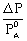= XB.

In above discussion B is a non volatile solute and A is pure liquid. Let us consider

## Lowering in Vapour Pressure Assignment Help By Online Tutoring and Guided Sessions at AssignmentHelp.Net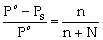(where ‘n’ and ‘N’ are moles of solute and solvent respectively).

For dilute solutions n << N, hence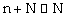\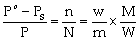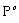= Vapour pressure of pure solvent

PS = Vapour pressure of solutions

n = Moles of solute

N = Moles of solvent

W = Mass of solvent in grams

m = Molecular mass of solute

w = Mass of solute in grams

M = Molecular mass of solvent

### Assignment Help For Lowering in Vapour Pressure

Assignmenthelp.net provides best Online Assignment Help service in Lowering in Vapour Pressure for all standards. Our Tutor provide their high quality and optimized Tutorial help to fulfill all kind of need of Students.